### Creating Line Printer Plots

Subsections:

When ODS Graphics is disabled, the PLOTS option in the PROC UNIVARIATE statement provides up to four diagnostic line printer plots to examine the data distribution. These plots are the stem-and-leaf plot or horizontal bar chart, the box plot, the normal probability plot, and the side-by-side box plots. If you specify the WEIGHT statement, PROC UNIVARIATE provides a weighted histogram, a weighted box plot based on the weighted quantiles, and a weighted normal probability plot.

Note that these plots are a legacy feature of the UNIVARIATE procedure in earlier versions of SAS. They predate the addition of the CDFPLOT, HISTOGRAM, PPPLOT, PROBPLOT, and QQPLOT statements, which provide high-resolution graphics displays. Also note that line printer plots requested with the PLOTS option are mainly intended for use with the ODS LISTING destination. See Example 4.5.

#### Stem-and-Leaf Plot

The first plot in the output is either a stem-and-leaf plot (Tukey, 1977) or a horizontal bar chart. If any single interval contains more than 49 observations, the horizontal bar chart appears. Otherwise, the stem-and-leaf plot appears. The stem-and-leaf plot is like a horizontal bar chart in that both plots provide a method to visualize the overall distribution of the data. The stem-and-leaf plot provides more detail because each point in the plot represents an individual data value.

To change the number of stems that the plot displays, use PLOTSIZE= to increase or decrease the number of rows. Instructions that appear below the plot explain how to determine the values of the variable. If no instructions appear, you multiply Stem.Leaf by 1 to determine the values of the variable. For example, if the stem value is 10 and the leaf value is 1, then the variable value is approximately 10.1. For the stem-and-leaf plot, the procedure rounds a variable value to the nearest leaf. If the variable value is exactly halfway between two leaves, the value rounds to the nearest leaf with an even integer value. For example, a variable value of 3.15 has a stem value of 3 and a leaf value of 2.

#### Box Plot

The box plot, also known as a schematic box plot, appears beside the stem-and-leaf plot. Both plots use the same vertical scale. The box plot provides a visual summary of the data and identifies outliers. The bottom and top edges of the box correspond to the sample 25th (Q1) and 75th (Q3) percentiles. The box length is one interquartile range (Q3 – Q1). The center horizontal line with asterisk endpoints corresponds to the sample median. The central plus sign (+) corresponds to the sample mean. If the mean and median are equal, the plus sign falls on the line inside the box. The vertical lines that project out from the box, called whiskers, extend as far as the data extend, up to a distance of 1.5 interquartile ranges. Values farther away are potential outliers. The procedure identifies the extreme values with a zero or an asterisk (*). If zero appears, the value is between 1.5 and 3 interquartile ranges from the top or bottom edge of the box. If an asterisk appears, the value is more extreme.

Note: To produce box plots that use high-resolution graphics, use the BOXPLOT procedure in SAS/STAT software. See Chapter 28: The BOXPLOT Procedure in SAS/STAT User's Guide.

#### Normal Probability Plot

The normal probability plot plots the empirical quantiles against the quantiles of a standard normal distribution. Asterisks (*) indicate the data values. The plus signs (+) provide a straight reference line that is drawn by using the sample mean and standard deviation. If the data are from a normal distribution, the asterisks tend to fall along the reference line. The vertical coordinate is the data value, and the horizontal coordinate is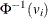where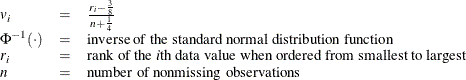For a weighted normal probability plot, theth ordered observation is plotted againstwhere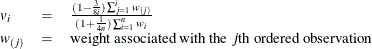When each observation has an identical weight,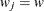, the formula for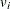reduces to the expression forin the unweighted normal probability plot: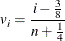When the value of VARDEF= is WDF or WEIGHT, a reference line with intercept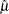and slope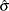is added to the plot. When the value of VARDEF= is DF or N, the slope is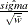where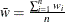is the average weight.

When each observation has an identical weight and the value of VARDEF= is DF, N, or WEIGHT, the reference line reduces to the usual reference line with intercept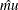and slopein the unweighted normal probability plot.

If the data are normally distributed with meanand standard deviation, and each observation has an identical weight, then the points on the plot should lie approximately on a straight line. The intercept for this line is. The slope iswhen VARDEF= is WDF or WEIGHT, and the slope is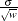when VARDEF= is DF or N.

Note: To produce high-resolution probability plots, use the PROBPLOT statement in PROC UNIVARIATE; see the section PROBPLOT Statement.

#### Side-by-Side Box Plots

When you use a BY statement with the PLOT option, PROC UNIVARIATE produces side-by-side box plots, one for each BY group. The box plots (also known as schematic plots) use a common scale that enables you to compare the data distribution across BY groups. This plot appears after the univariate analyses of all BY groups. Use the NOBYPLOT option to suppress this plot.

Note: To produce high-resolution side-by-side box plots, use the BOXPLOT procedure in SAS/STAT software. See Chapter 28: The BOXPLOT Procedure in SAS/STAT User's Guide.